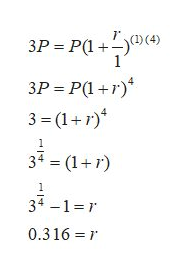Solve the problem. Round your answer to three decimals. What annual rate of interest is required to triple an investment in 4 years?

Question

Solve the problem. Round your answer to three decimals.

What annual rate of interest is required to triple an investment in 4 years?

Step 1

We use the following compound interest formula, where

A: = future value of the deposit,

P: = principal or amount of money deposited,

r: = annual interest rate in decimal form,

n: = number of times compounded per year,

t: = total time in years.

Step 2

Now as give in question,

A = 3P, t = 4, n = 1,

Substi...help_outlineImage Transcriptionclose3P P(1 1 3P P(1+) 3-(1+r) 34 (1+) 34 -1 r 0.316 fullscreen

Want to see the full answer?

See Solution

Want to see this answer and more?

Our solutions are written by experts, many with advanced degrees, and available 24/7

See Solution
Tagged in

Other